Search by Topic

Resources tagged with Maths Supporting SET similar to Tangled Trig Graphs:

Filter by: Content type:
Age range:
Challenge level:

There are 89 results

Broad Topics > Applications > Maths Supporting SETScientific Curves

Age 16 to 18 Challenge Level:

Can you sketch these difficult curves, which have uses in mathematical modelling?Whose Line Graph Is it Anyway?

Age 16 to 18 Challenge Level:

Which line graph, equations and physical processes go together?Real-life Equations

Age 16 to 18 Challenge Level:

Here are several equations from real life. Can you work out which measurements are possible from each equation?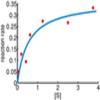Curve Fitter 2

Age 16 to 18 Challenge Level:

Can you construct a cubic equation with a certain distance between its turning points?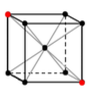Crystal Symmetry

Age 16 to 18 Challenge Level:

Use vectors and matrices to explore the symmetries of crystals.Taking Trigonometry Series-ly

Age 16 to 18 Challenge Level:

Look at the advanced way of viewing sin and cos through their power series.What Do Functions Do for Tiny X?

Age 16 to 18 Challenge Level:

Looking at small values of functions. Motivating the existence of the Taylor expansion.Fix Me or Crush Me

Age 16 to 18 Challenge Level:

Can you make matrices which will fix one lucky vector and crush another to zero?Equation Matcher

Age 16 to 18 Challenge Level:

Can you match these equations to these graphs?Back Fitter

Age 14 to 16 Challenge Level:

10 graphs of experimental data are given. Can you use a spreadsheet to find algebraic graphs which match them closely, and thus discover the formulae most likely to govern the underlying processes?Building Approximations for Sin(x)

Age 16 to 18 Challenge Level:

Build up the concept of the Taylor seriesWhat's That Graph?

Age 14 to 16 Challenge Level:

Can you work out which processes are represented by the graphs?Square Pair

Age 16 to 18 Challenge Level:

Explore the shape of a square after it is transformed by the action of a matrix.Nine Eigen

Age 16 to 18 Challenge Level:

Explore how matrices can fix vectors and vector directions.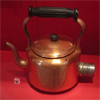Electric Kettle

Age 14 to 16 Challenge Level:

Explore the relationship between resistance and temperature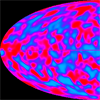Big and Small Numbers in Physics

Age 14 to 16 Challenge Level:

Work out the numerical values for these physical quantities.Global Warming

Age 14 to 16 Challenge Level:

How much energy has gone into warming the planet?Immersion

Age 14 to 16 Challenge Level:

Various solids are lowered into a beaker of water. How does the water level rise in each case?Aim High

Age 16 to 18 Challenge Level:

How do you choose your planting levels to minimise the total loss at harvest time?The Wrong Stats

Age 16 to 18 Challenge Level:

Why MUST these statistical statements probably be at least a little bit wrong?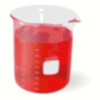Brimful 2

Age 16 to 18 Challenge Level:

Which of these infinitely deep vessels will eventually full up?Operating Machines

Age 16 to 18 Challenge Level:

What functions can you make using the function machines RECIPROCAL and PRODUCT and the operator machines DIFF and INT?Big and Small Numbers in the Living World

Age 11 to 16 Challenge Level:

Work with numbers big and small to estimate and calculate various quantities in biological contexts.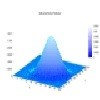Pdf Matcher

Age 16 to 18 Challenge Level:

Which pdfs match the curves?Approximately Certain

Age 14 to 18 Challenge Level:

Estimate these curious quantities sufficiently accurately that you can rank them in order of size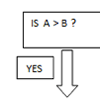Mystery Procedure

Age 14 to 16 Challenge Level:

Can you work out what this procedure is doing?Constantly Changing

Age 14 to 16 Challenge Level:

Many physical constants are only known to a certain accuracy. Explore the numerical error bounds in the mass of water and its constituents.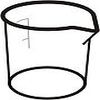Brimful

Age 16 to 18 Challenge Level:

Can you find the volumes of the mathematical vessels?Vector Walk

Age 14 to 18 Challenge Level:

Starting with two basic vector steps, which destinations can you reach on a vector walk?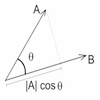Cross with the Scalar Product

Age 16 to 18 Challenge Level:

Explore the meaning of the scalar and vector cross products and see how the two are related.Polygon Walk

Age 16 to 18 Challenge Level:

Go on a vector walk and determine which points on the walk are closest to the origin.Perfect Eclipse

Age 14 to 16 Challenge Level:

Use trigonometry to determine whether solar eclipses on earth can be perfect.Transformations for 10

Age 16 to 18 Challenge Level:

Explore the properties of matrix transformations with these 10 stimulating questions.Matrix Meaning

Age 16 to 18 Challenge Level:

Explore the meaning behind the algebra and geometry of matrices with these 10 individual problems.Big and Small Numbers in Chemistry

Age 14 to 16 Challenge Level:

Get some practice using big and small numbers in chemistry.Pdf Stories

Age 16 to 18 Challenge Level:

Invent scenarios which would give rise to these probability density functions.Stirling Work

Age 16 to 18 Challenge Level:

See how enormously large quantities can cancel out to give a good approximation to the factorial function.Integration Matcher

Age 16 to 18 Challenge Level:

Can you match the charts of these functions to the charts of their integrals?Maths Filler 2

Age 14 to 16 Challenge Level:

Can you draw the height-time chart as this complicated vessel fills with water?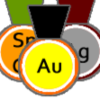Alternative Record Book

Age 14 to 18 Challenge Level:

In which Olympic event does a human travel fastest? Decide which events to include in your Alternative Record Book.Quorum-sensing

Age 16 to 18 Short Challenge Level:

This problem explores the biology behind Rudolph's glowing red nose.Biology Measurement Challenge

Age 14 to 16 Challenge Level:

Analyse these beautiful biological images and attempt to rank them in size order.Far Horizon

Age 14 to 16 Challenge Level:

An observer is on top of a lighthouse. How far from the foot of the lighthouse is the horizon that the observer can see?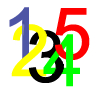Track Design

Age 14 to 16 Challenge Level:

Where should runners start the 200m race so that they have all run the same distance by the finish?Over-booking

Age 16 to 18 Challenge Level:

The probability that a passenger books a flight and does not turn up is 0.05. For an aeroplane with 400 seats how many tickets can be sold so that only 1% of flights are over-booked?Perspective Drawing

Age 11 to 16 Challenge Level:

Explore the properties of perspective drawing.Spherical Triangles on Very Big Spheres

Age 16 to 18 Challenge Level:

Shows that Pythagoras for Spherical Triangles reduces to Pythagoras's Theorem in the plane when the triangles are small relative to the radius of the sphere.Differential Equation Matcher

Age 16 to 18 Challenge Level:

Match the descriptions of physical processes to these differential equations.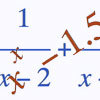Root Hunter

Age 16 to 18 Challenge Level:

In this short problem, try to find the location of the roots of some unusual functions by finding where they change sign.Scale Invariance

Age 16 to 18 Challenge Level:

By exploring the concept of scale invariance, find the probability that a random piece of real data begins with a 1.